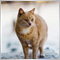• Overview
• Reviews (2)

# Cross Trend Lines

Cross  Trend Lines  indicator trend indicator of find. This indicator, an array of works by using a series of indicator and algorithm.

Uses two lines to find trends. The intersection of this line means that the start of the trend. These lines are green, tend to rise. Red lines indicate the downward trend.

Use of this indicator is easy to understand. This indicator on its own or you can use other indicators that are appropriate for the strategy.

### Suggestions and Features

• Starts a new trend at the intersection of the lines
• Green means the rising trend lines.
• If the decline trend lines refers to red.
• Long as you want is short and you can adjust the audio settings.
• The method property, you can set the method you want with.

### Parameters

• Short_Length - The short trend length setting is done from here.
• Short_Method - The short trend method setting is done from here. Methods: 0= Simple, 1= Exponential, 2= Smoothed, 3= Linear weighted.
• Long_Length - The long trend length setting is done from here.
• Long_Method - The long trend method is set from here. Methods: 0= Simple, 1= Exponential, 2= Smoothed, 3= Linear weighted.
• Volume_Length - Volume length sensitivity setting is done from here.
• Volume_Method - Volume method sensitivity setting is done from here. Methods: 0= Simple, 1= Exponential, 2= Smoothed, 3= Linear weighted.53
2019.01.29 23:00

User didn't leave any comment to the rating8565
2018.12.01 13:43

Good tool, thank you very much